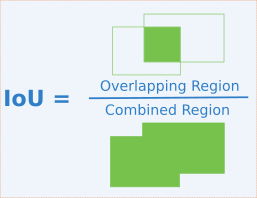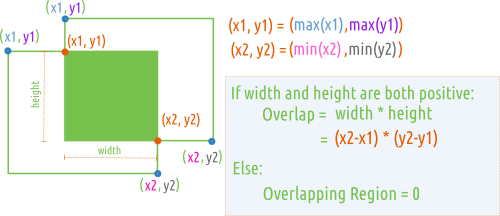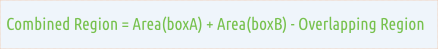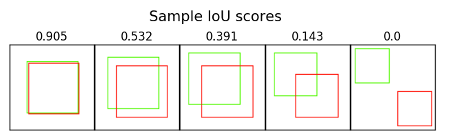# Intersect over Union (IoU)

Intersect over Union (IoU) is a metric that allows us to evaluate how similar our predicted bounding box is to the ground truth bounding box. The idea is that we want to compare the ratio of the area where the two boxes overlap to the total combined area of the two boxes.

The formula for calculating IoU is as follows.### Calculating Overlapping Region.### Calculating Combined Region.## Interpreting IoU scores

IoU is quite intuitive to interpret. A score of 1 means that the predicted bounding box precisely matches the ground truth bounding box. A score of 0 means that the predicted and true bounding box do not overlap at all.## Sample code

### Simple Version

The following is a simple Python implementation which calculates the IoU for two boxes.

```def get_iou(a, b, epsilon=1e-5):
""" Given two boxes `a` and `b` defined as a list of four numbers:
[x1,y1,x2,y2]
where:
x1,y1 represent the upper left corner
x2,y2 represent the lower right corner
It returns the Intersect of Union score for these two boxes.

Args:
a:          (list of 4 numbers) [x1,y1,x2,y2]
b:          (list of 4 numbers) [x1,y1,x2,y2]
epsilon:    (float) Small value to prevent division by zero

Returns:
(float) The Intersect of Union score.
"""
# COORDINATES OF THE INTERSECTION BOX
x1 = max(a, b)
y1 = max(a, b)
x2 = min(a, b)
y2 = min(a, b)

# AREA OF OVERLAP - Area where the boxes intersect
width = (x2 - x1)
height = (y2 - y1)
# handle case where there is NO overlap
if (width<0) or (height <0):
return 0.0
area_overlap = width * height

# COMBINED AREA
area_a = (a - a) * (a - a)
area_b = (b - b) * (b - b)
area_combined = area_a + area_b - area_overlap

# RATIO OF AREA OF OVERLAP OVER COMBINED AREA
iou = area_overlap / (area_combined+epsilon)
return iou
```

### Batch Version

The following is a Python implementation which calculates the IoU for batches of bounding boxes.

```def batch_iou(a, b, epsilon=1e-5):
""" Given two arrays `a` and `b` where each row contains a bounding
box defined as a list of four numbers:
[x1,y1,x2,y2]
where:
x1,y1 represent the upper left corner
x2,y2 represent the lower right corner
It returns the Intersect of Union scores for each corresponding
pair of boxes.

Args:
a:          (numpy array) each row containing [x1,y1,x2,y2] coordinates
b:          (numpy array) each row containing [x1,y1,x2,y2] coordinates
epsilon:    (float) Small value to prevent division by zero

Returns:
(numpy array) The Intersect of Union scores for each pair of bounding
boxes.
"""
# COORDINATES OF THE INTERSECTION BOXES
x1 = np.array([a[:, 0], b[:, 0]]).max(axis=0)
y1 = np.array([a[:, 1], b[:, 1]]).max(axis=0)
x2 = np.array([a[:, 2], b[:, 2]]).min(axis=0)
y2 = np.array([a[:, 3], b[:, 3]]).min(axis=0)

# AREAS OF OVERLAP - Area where the boxes intersect
width = (x2 - x1)
height = (y2 - y1)

# handle case where there is NO overlap
width[width < 0] = 0
height[height < 0] = 0

area_overlap = width * height

# COMBINED AREAS
area_a = (a[:, 2] - a[:, 0]) * (a[:, 3] - a[:, 1])
area_b = (b[:, 2] - b[:, 0]) * (b[:, 3] - b[:, 1])
area_combined = area_a + area_b - area_overlap

# RATIO OF AREA OF OVERLAP OVER COMBINED AREA
iou = area_overlap / (area_combined + epsilon)
return iou
```